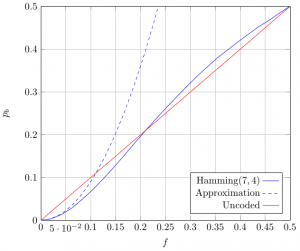MA331 Information Theory and Channel Coding

The objective of this course is to understand how we can transmit a message in-between a transmitter and a receiver spatially (or temporally) separated. Especially, how we can use coding to realize an error free transmission over noisy channels. This beautiful result has been predicted by C. E. Shannon and relies on two distinct operations which are source coding and channel coding. This course is an introduction to information theory and describe the main algorithms in both source coding and channel coding. This course is related to SC311 Wireless Communications but tackle the communication problem mainly at the bit level.

This course is composed of 3 parts. Slides (in french) for each part can be downloaded here:

Introduction

Information Theory

Source Coding

Channel Coding

This objective of this lab is to allow you to evaluate the performance of classical telecommunication system by simulating the transmission of a large quantity of random data (Monte Carlo method). A special focus is placed on the performance of forward error correcting codes over different channels. The original subject of this lab is based on Matlab (or Octave) and is available here (in French) where the objective is to estimate the performance of the classical Hamming code $H(7,4)$over the BSC channel of probability $f$.

This wiki presents a different approach based on the IT++ library which allows to generalize the results for any forward error correcting code and any channel.

IT++

IT++ is a C++ library designed to evaluate the performance of different telecommunications systems. Several forward error correction codes have been implemented and can easily be characterized and compared. All the results presented during the course have been obtained using this library.

Hamming Code

• After installing the library (which is maybe the hardest step of this lab), create a file main.cpp and copy the following program.
#include <itpp/itcomm.h>

using namespace itpp;
using std::cout;
using std::endl;

int main()
{
bvec bits, ebits, dbits;

Hamming_Code hc(3);

RNG_randomize();

bits = randb(4);              // Generate 4 random bits

ebits = hc.encode(bits);      // Encode with H(7,4)

dbits = hc.decode(ebits);     // Decode with H(7,4)

cout << "bits = " << bits << endl;
cout << "ebits = " << ebits << endl;
cout << "dbits = " << dbits << endl;

return 0;
}

The program can be built with the following command:

$g++ pkg-config --cflags itpp -o main main.cpp pkg-config --libs itpp If sucessfull, this command produces the binary file main which can be directly executed by typing:$ ./main
• Determine the generator matrix $G$of the Hamming code $H(7,4)$.
• Modify the encoded vector by adding 1 error on the codeword and observe the decoded codeword.
• Same question with 2 errors.

Performance over BSC channel

#include <itpp/itcomm.h>

#define N 500000

using namespace itpp;
using std::cout;
using std::endl;

int main()
{
bvec bits, ebits, rbits, dbits;
vec f = linspace(0.0, 0.5, 20);
vec ber(p.length());

BSC bsc;
BERC berc;
Hamming_Code hc(3);
it_file ff;

RNG_randomize();

for (int i = 0; i < p.length(); i++)
{
bsc.set_prob(f(i));         // Set the error prob of the BSC
bits = randb(N);            // Generate N random bits
ebits = hc.encode(bits);    // Encode with H(7,4)
rbits = bsc(ebits);         // Transmit over BSC
dbits = hc.decode(rbits);   // Decode with H(7,4)
berc.clear();               // Reset the counter
berc.count(bits, dbits);    // Count the errors
ber(i) = berc.get_errorrate();
}
cout << "BER = " << ber << endl;
ff.open("res.it");            // Save the results
ff << Name("f") << f;
ff << Name("ber") << ber;
ff.close();

return 0;
}

Execution takes few seconds and display the BER for the different probabilities $f$of the BSC channel. Results are also saved in the file res.it and can be easily displayed using the itload.m script using Matlab (or Octave):Performance of the Hamming code H(7,4) over BSC of probability $f$.
{\begin{aligned}p_{B}&=\sum _{i=2}^{7}{\binom {7}{i}}f^{i}(1-f)^{7-i}\\&\approx {\binom {7}{2}}f^{2}\\&\approx 21f^{2}\\\end{aligned}}$p_{b}\approx {\frac {3}{7}}p_{B}\approx 9f^{2}$